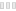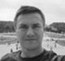# Math Mechanixs

CleanA review by AndrewThe invention of calculators has made work easier. Math Mechanixs is a tool that enables you to perform mathematical calculations. It is a widely used tool for all professions which carryout mathematics calculations like engineers, students, scientists. It caters for all mathematicians. It runs on a math editor and not text editor. The math editor allows you to write the mathematical expression, the interface is remotely integrated to allow you calculate all the mathematical calculations and solutions given and the steps used to reach to the solution. It has graphical interpretation in 2D and 3D presentation to take care of graphical solutions. It is a comprehensive and powerful tool which supports geometry, calculus, algebra, quadratic expressions among other mathematical concepts.

The interface is intuitive and interactive though it needs a little expertise to allow it run smoothly. Each function and expressions are run on separate windows making tracking of all operations of all mathematical functions easily accessible. The software can run many mathematical calculations within seconds. The multiple functions are run simultaneously on different tabs. It is a suitable tool for both simple and complex and simple mathematical expressions.

It is part from mathematics category and is licensed as shareware for Windows 32-bit and 64-bit platform and can be used as a free trial until the trial period will end. The Math Mechanixs demo is available to all software users as a free download with potential restrictions compared with the full version.

 Share Give a rating(0 votes, average: 0.00 out of 5)login to voteLoading... Author Math Mechanixs, LLC. Last Updated On April 18, 2017 Runs on Windows 10 / Windows 8 / Windows 7 / Windows Vista / XP Total downloads 322 License Free to try (Shareware) File size 17,59 MB Filename mathmechsetup.exe

### Similar programsxFunc
Free